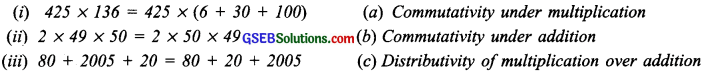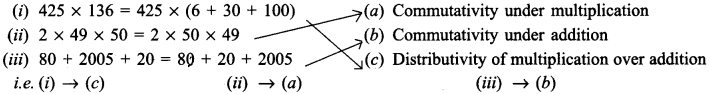# GSEB Solutions Class 6 Maths Chapter 2 Whole Numbers Ex 2.2

Gujarat Board GSEB Textbook Solutions Class 6 Maths Chapter 2 Whole Numbers Ex 2.2 Textbook Questions and Answers.

## Gujarat Board Textbook Solutions Class 6 Maths Chapter 2 Whole Numbers Ex 2.2

Question 1.
Find the sum by suitable rearrangement:
(a) 837 + 208 + 363
(b) 1962 + 453 + 1538 + 647
Solution:
(a) 837 + 208 + 363 208 + (363 + 837)
= 208 + (1200) = 1408

(b) 1962 + 453 + 1538 + 647
= (1962 + 1538) + (453 + 647)
= (3500) + (1100)
= 3500 + 1100 = 4600Question 2.
Find the product by suitable rearrangement:
(a) 2 x 1768 x 50
(b) 4 x 166 x 25
(c) 8 x 291 x 125
(d) 625 x 279 x 16
(e) 285 x 5 x 60
(f) 125 x 40 x 8 x 25
Solution:
(a) 2 x 1768 x 50 = (2 x 50) x 1768
= 100 x 1768 = 176800

(b) 4 x 166 x 25 = (4 x 25) x 166
= 1oo x 166 = 16600

(c) 8 x 291 x 125 = (8 x 125) x 291
= (1000) x 291 = 291000

(d) 625 x 279 X 16 = (625 x 16) x 279
= (10000) x 279 = 2790000

(e) 285 x 5 x 60 = 285 x (5 x 60) = 285 x 300
= (285 x 3) x 1oo = 855 x 100 = 85500

(f) 125 x 40 x 8 x 25 = (125 x 8) x (40 x 25)
= (1000) x (1000) = 1000000Question 3.
Find the value of the following:
(a) 297 x 17 + 297 x 3
(b) 54279 x 92 + 8 x 54279
(c) 81265 x 169 – 81265 x 69
(d) 3845 x 5 x 782 + 769 x 25 x 218
Solution:
(a) 297 x 17 + 297 x 3 = 297 [17 + 3] = 297 
= 297 x 20 = 5940

(b) 54279 x 92 + 8 x 54279 = 54279 [92 + 8]
= 54279  = 54279 x 100 = 5427900

(c) 81265 x 169 – 81265 x 69 = 81265 [169 – 69]
= 81265  = 81265 x 100 = 8126500

(d) 3845 x 5×782 + 769 x 25 x 218
(25 = 5 x 5)
= 3845 x 5 x 782 + (769 x 5) x 5 x 218
= 3845 x 5 x 782 + 3845 x 5 x 218
= 3845 x 5 x [782 + 218]
= 3845 x 5 x 
= 19225 x 1000 = 19225000Question 4.
Find the product using suitable properties.
(a) 738 x 103
(b) 854 x 102
(e) 258 x 1008
(d) 1005 x 168
Solution:
(a) 738 x 103 738 x [100 + 3]
= [738 100] + [738 x 3]
= 73800 + 2214 = 76014

(b) 854 x 102 = 854 x [100 + 2]
= (854 x 100) + (854 X 2)
= 85400 + 1708 = 87108

(c) 258 x 1008 = 258 x [1000 + 8]
= (258 x 1000) + (258 x 8)
= 258000 + 2064 = 260064

(d) 1005 x 168 = [1000 + 5] x 168
= (1000 x 168) + (5 x 168)
= 168000 + 840 = 168840Question 5.
A taxi driver filled his car petrol tank with 40 litres of petrol on Monday. The next day, he filled the tank with 50 litres of petrol. If the petrol costs 44 per litre, how much did he spend in all on petrol?
Solution:
Quantity of petrol filled on Monday = 40 litres
Quantity of petrol filled on the next day = 50 litres
Per litre cost of petrol = ₹ 44
Total cost of petrol for the two days
= ₹ 44 x [40 + 50]
= ₹ [44 x 50] + [44 x 40]
= ₹ 2200 + ₹ 1760 = ₹ 3960

Question 6.
A vendor supplies 32 litres of milk to a hotel in the morning and 68 litres of milk in the evening. If the milk costs ₹ 15 per litre, how much money is due to the vendor per day?
Solution:
Milk supplied in the
Morning = 32 litres
Evening = 68 litres
Cost of milk = ₹ 15 per litre
Total cost of milk per day
= ₹ 15 x [32 – 4 – 68] = ₹ 15 x 100
= ₹ 1500
Money is due to the vendor per day = ₹ 1500Question 7.
Match the following:Solution: